x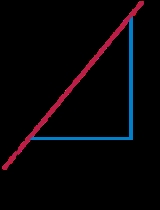SlopeEncyclopedia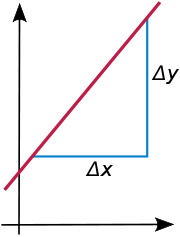In mathematics
Mathematics
Mathematics is the study of quantity, space, structure, and change. Mathematicians seek out patterns and formulate new conjectures. Mathematicians resolve the truth or falsity of conjectures by mathematical proofs, which are arguments sufficient to convince other mathematicians of their validity...

, the slope or gradient of a line
Line (mathematics)
The notion of line or straight line was introduced by the ancient mathematicians to represent straight objects with negligible width and depth. Lines are an idealization of such objects...

describes its steepness, incline, or grade. A higher slope value indicates a steeper incline.

The slope is defined as the ratio of the "rise" divided by the "run" between two points on a line, or in other words, the ratio of the altitude change to the horizontal distance between any two points on the line. Given two points (x1,y1) and (x2,y2) on a line, the slope m of the line is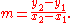Through differential calculus
Differential calculus
In mathematics, differential calculus is a subfield of calculus concerned with the study of the rates at which quantities change. It is one of the two traditional divisions of calculus, the other being integral calculus....

, one can calculate the slope of the tangent line
Tangent
In geometry, the tangent line to a plane curve at a given point is the straight line that "just touches" the curve at that point. More precisely, a straight line is said to be a tangent of a curve at a point on the curve if the line passes through the point on the curve and has slope where f...

to a curve
Curve
In mathematics, a curve is, generally speaking, an object similar to a line but which is not required to be straight...

at a point.

In vector calculus, the gradient of a scalar field is a vector field that points in the direction of the greatest rate of increase of the scalar field, and whose magnitude is the greatest rate of change....

s in geography
Geography
Geography is the science that studies the lands, features, inhabitants, and phenomena of Earth. A literal translation would be "to describe or write about the Earth". The first person to use the word "geography" was Eratosthenes...

and civil engineering
Civil engineering
Civil engineering is a professional engineering discipline that deals with the design, construction, and maintenance of the physical and naturally built environment, including works like roads, bridges, canals, dams, and buildings...

. Through trigonometry
Trigonometry
Trigonometry is a branch of mathematics that studies triangles and the relationships between their sides and the angles between these sides. Trigonometry defines the trigonometric functions, which describe those relationships and have applicability to cyclical phenomena, such as waves...

, the grade m of a road is related to its angle of incline θ by## Definition

The slope of a line in the plane containing the x and y axes is generally represented by the letter m, and is defined as the change in the y coordinate divided by the corresponding change in the x coordinate, between two distinct points on the line. This is described by the following equation: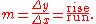(The delta symbol, "Δ", is commonly used in mathematics to mean "difference" or "change".)

Given two points (x1,y1) and (x2,y2), the change in x from one to the other is (run), while the change in y is (rise). Substituting both quantities into the above equation generates the formula:## Examples

Suppose a line runs through two points: P = (1, 2) and Q = (13, 8). By dividing the difference in y-coordinates by the difference in x-coordinates, one can obtain the slope of the line: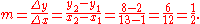As another example, consider a line which runs through the points (4, 15) and (3, 21). Then, the slope of the line is## Geometry

The larger the absolute value of a slope, the steeper the line. A horizontal line has slope 0, a 45° rising line has a slope of +1, and a 45° falling line has a slope of −1. A vertical line's slope is undefined meaning it has "no slope."

The angle θ a line makes with the positive x axis is closely related to the slope m via the tangent function: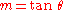and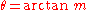(see trigonometry
Trigonometry
Trigonometry is a branch of mathematics that studies triangles and the relationships between their sides and the angles between these sides. Trigonometry defines the trigonometric functions, which describe those relationships and have applicability to cyclical phenomena, such as waves...

).

Two lines are parallel if and only if their slopes are equal and they are not coincident or if they both are vertical and therefore have undefined slopes. Two lines are perpendicular
Perpendicular
In geometry, two lines or planes are considered perpendicular to each other if they form congruent adjacent angles . The term may be used as a noun or adjective...

if the product of their slopes is −1 or one has a slope of 0 (a horizontal line) and the other has an undefined slope (a vertical line). Also, another way to determine a perpendicular line is to find the slope of one line and then to get its reciprocal and then reversing its positive or negative sign (e.g. a line perpendicular to a line of slope  −2 is +1/2).

### Slope of a road or railway

Grade separation is the method of aligning a junction of two or more transport axes at different heights so that they will not disrupt the traffic flow on other transit routes when they cross each other. The composition of such transport axes does not have to be uniform; it can consist of a...

There are two common ways to describe how steep a road
A road is a thoroughfare, route, or way on land between two places, which typically has been paved or otherwise improved to allow travel by some conveyance, including a horse, cart, or motor vehicle. Roads consist of one, or sometimes two, roadways each with one or more lanes and also any...

Rail tracks
The track on a railway or railroad, also known as the permanent way, is the structure consisting of the rails, fasteners, sleepers and ballast , plus the underlying subgrade...

is. One is by the angle in degrees, and the other is by the slope in a percentage. See also mountain railway
Mountain railway
A mountain railway is a railway that ascends and descends a mountain slope that has a steep grade. Such railways can use a number of different technologies to overcome the steepness of the grade...

and rack railway
Rack railway
A rack-and-pinion railway is a railway with a toothed rack rail, usually between the running rails. The trains are fitted with one or more cog wheels or pinions that mesh with this rack rail...

. The formulae for converting a slope as a percentage into an angle in degrees and vice versa are: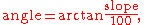and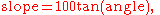where angle is in degrees and the trigonometric functions operate in degrees. For example, a 100%
Percent sign
The percent sign is the symbol used to indicate a percentage .Related signs include the permille sign ‰ and the permyriad sign , which indicate that a number is divided by one thousand or ten thousand respectively...

or 1000‰ slope is 45°.

A third way is to give one unit of rise in say 10, 20, 50 or 100 horizontal units, e.g. 1:10. 1:20, 1:50 or 1:100 (etc.).

## Algebra

If y is a linear function
Linear function
In mathematics, the term linear function can refer to either of two different but related concepts:* a first-degree polynomial function of one variable;* a map between two vector spaces that preserves vector addition and scalar multiplication....

of x, then the coefficient of x is the slope of the line created by plotting the function. Therefore, if the equation of the line is given in the form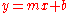then m is the slope. This form of a line's equation is called the slope-intercept form, because b can be interpreted as the y-intercept
Y-intercept
In coordinate geometry, using the common convention that the horizontal axis represents a variable x and the vertical axis represents a variable y, a y-intercept is a point where the graph of a function or relation intersects with the y-axis of the coordinate system...

of the line, the y-coordinate where the line intersects the y-axis.

If the slope m of a line and a point (x1,y1) on the line are both known, then the equation of the line can be found using the point-slope formula: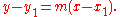For example, consider a line running through the points (2,8) and (3,20). This line has a slope, m, of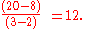One can then write the line's equation, in point-slope form: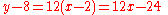or:The slope of the line defined by the linear equation
Linear equation
A linear equation is an algebraic equation in which each term is either a constant or the product of a constant and a single variable....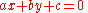is:.

## Calculus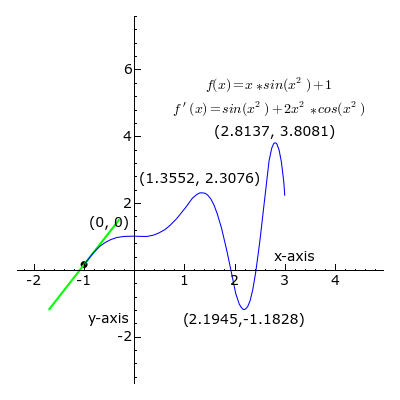The concept of a slope is central to differential calculus
Differential calculus
In mathematics, differential calculus is a subfield of calculus concerned with the study of the rates at which quantities change. It is one of the two traditional divisions of calculus, the other being integral calculus....

. For non-linear functions, the rate of change varies along the curve. The derivative
Derivative
In calculus, a branch of mathematics, the derivative is a measure of how a function changes as its input changes. Loosely speaking, a derivative can be thought of as how much one quantity is changing in response to changes in some other quantity; for example, the derivative of the position of a...

of the function at a point is the slope of the line tangent
Tangent
In geometry, the tangent line to a plane curve at a given point is the straight line that "just touches" the curve at that point. More precisely, a straight line is said to be a tangent of a curve at a point on the curve if the line passes through the point on the curve and has slope where f...

to the curve at the point, and is thus equal to the rate of change of the function at that point.

If we let Δx and Δy be the distances (along the x and y axes, respectively) between two points on a curve, then the slope given by the above definition,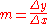,

is the slope of a secant line
Secant line
A secant line of a curve is a line that intersects two points on the curve. The word secant comes from the Latin secare, to cut.It can be used to approximate the tangent to a curve, at some point P...

to the curve. For a line, the secant between any two points is the line itself, but this is not the case for any other type of curve.

For example, the slope of the secant intersecting y = x2 at (0,0) and (3,9) is 3. (The slope of the tangent at is also 3—a consequence of the mean value theorem.)

By moving the two points closer together so that Δy and Δx decrease, the secant line more closely approximates a tangent line to the curve, and as such the slope of the secant approaches that of the tangent. Using differential calculus
Differential calculus
In mathematics, differential calculus is a subfield of calculus concerned with the study of the rates at which quantities change. It is one of the two traditional divisions of calculus, the other being integral calculus....

, we can determine the limit
Limit of a function
In mathematics, the limit of a function is a fundamental concept in calculus and analysis concerning the behavior of that function near a particular input....

, or the value that Δyx approaches as Δy and Δx get closer to zero; it follows that this limit is the exact slope of the tangent. If y is dependent on x, then it is sufficient to take the limit where only Δx approaches zero. Therefore, the slope of the tangent is the limit of Δyx as Δx approaches zero. We call this limit the derivative.

In vector calculus, the gradient of a scalar field is a vector field that points in the direction of the greatest rate of increase of the scalar field, and whose magnitude is the greatest rate of change....

is a generalization of the concept of slope for functions of more than one variable.
• Slope definitions
• Euclidean distance
Euclidean distance
In mathematics, the Euclidean distance or Euclidean metric is the "ordinary" distance between two points that one would measure with a ruler, and is given by the Pythagorean formula. By using this formula as distance, Euclidean space becomes a metric space...

• Theil–Sen estimator
Theil–Sen estimator
In non-parametric statistics, the Theil–Sen estimator, also known as Sen's slope estimator, slope selection, the single median method, or the Kendall robust line-fit method, is a method for robust linear regression that chooses the median slope among all lines through pairs of two-dimensional...

, a line with the median
Median
In probability theory and statistics, a median is described as the numerical value separating the higher half of a sample, a population, or a probability distribution, from the lower half. The median of a finite list of numbers can be found by arranging all the observations from lowest value to...

slope among a set of sample points